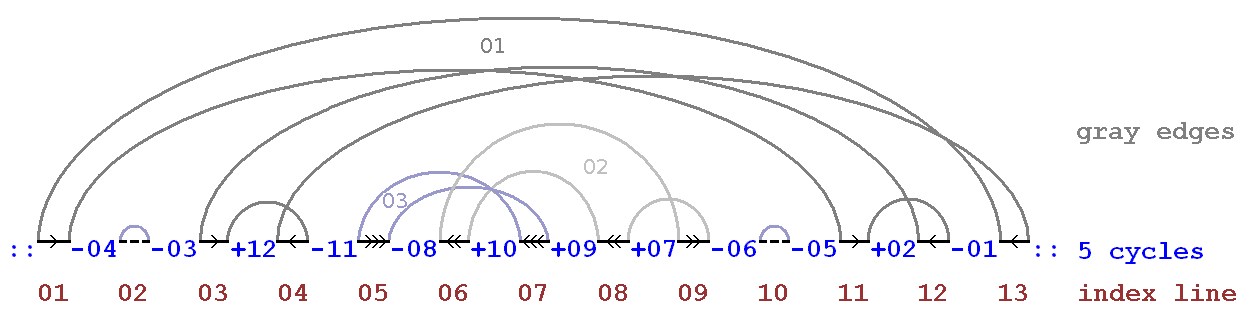# Cycle Sort Basic Information

suggest change

Cycle Sort is sorting algorithm which uses comparison sort that is theoretically optimal in terms of the total number of writes to original array, unlike any other in-place sorting algorithm. Cycle sort is unstable sorting algorithm. It is based on idea of permutation in which permutations are factored into cycles, which individually rotate and return a sorted output.

Example of Cycle Sort:Auxiliary Space: `O(1)`

Time Complexity: `O(n^2)`